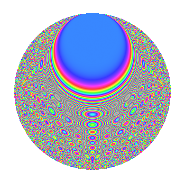# Properties

 Label 64.6.iLevel 64 Weight 6 Character orbit i Rep. character $$\chi_{64}(5,\cdot)$$ Character field $$\Q(\zeta_{16})$$ Dimension 312 Newform subspaces 1 Sturm bound 48 Trace bound 0

# Related objects

## Defining parameters

 Level: $$N$$ $$=$$ $$64 = 2^{6}$$ Weight: $$k$$ $$=$$ $$6$$ Character orbit: $$[\chi]$$ $$=$$ 64.i (of order $$16$$ and degree $$8$$) Character conductor: $$\operatorname{cond}(\chi)$$ $$=$$ $$64$$ Character field: $$\Q(\zeta_{16})$$ Newform subspaces: $$1$$ Sturm bound: $$48$$ Trace bound: $$0$$

## Dimensions

The following table gives the dimensions of various subspaces of $$M_{6}(64, [\chi])$$.

Total New Old
Modular forms 328 328 0
Cusp forms 312 312 0
Eisenstein series 16 16 0

## Trace form

 $$312q - 8q^{2} - 8q^{3} - 8q^{4} - 8q^{5} - 8q^{6} - 8q^{7} - 8q^{8} - 8q^{9} + O(q^{10})$$ $$312q - 8q^{2} - 8q^{3} - 8q^{4} - 8q^{5} - 8q^{6} - 8q^{7} - 8q^{8} - 8q^{9} - 8q^{10} - 8q^{11} - 8q^{12} - 8q^{13} - 8q^{14} - 8q^{15} - 8q^{16} - 8q^{17} - 8q^{18} - 8q^{19} - 8q^{20} - 8q^{21} + 13584q^{22} - 8q^{23} - 22048q^{24} - 8q^{25} - 25968q^{26} - 8q^{27} + 4352q^{28} - 8q^{29} + 64872q^{30} + 37152q^{32} + 12752q^{34} - 8q^{35} - 62328q^{36} - 8q^{37} - 69648q^{38} - 8q^{39} - 62368q^{40} - 8q^{41} + 46352q^{42} - 8q^{43} + 65504q^{44} - 8q^{45} - 8q^{46} - 8q^{47} - 8q^{48} - 8q^{49} - 137072q^{50} + 41752q^{51} + 73480q^{52} - 8q^{53} + 233272q^{54} - 220104q^{55} + 150912q^{56} - 8q^{57} + 25984q^{58} + 57912q^{59} - 197864q^{60} - 8q^{61} - 175384q^{62} + 317504q^{63} - 374888q^{64} - 16q^{65} - 254632q^{66} + 122312q^{67} - 7160q^{68} - 8q^{69} + 286936q^{70} - 287688q^{71} + 414064q^{72} - 8q^{73} + 377712q^{74} - 386504q^{75} + 268472q^{76} - 8q^{77} + 332656q^{78} + 355352q^{79} - 299872q^{80} - 8q^{81} - 503128q^{82} - 8q^{83} - 985048q^{84} - 8q^{85} - 721872q^{86} - 8q^{87} - 223288q^{88} - 8q^{89} + 284392q^{90} - 8q^{91} + 921264q^{92} + 1936q^{93} + 821272q^{94} + 1214336q^{96} + 957296q^{98} + 1936q^{99} + O(q^{100})$$

## Decomposition of $$S_{6}^{\mathrm{new}}(64, [\chi])$$ into newform subspaces

Label Dim. $$A$$ Field CM Traces $q$-expansion
$$a_2$$ $$a_3$$ $$a_5$$ $$a_7$$
64.6.i.a $$312$$ $$10.265$$ None $$-8$$ $$-8$$ $$-8$$ $$-8$$

## Hecke characteristic polynomials

There are no characteristic polynomials of Hecke operators in the database